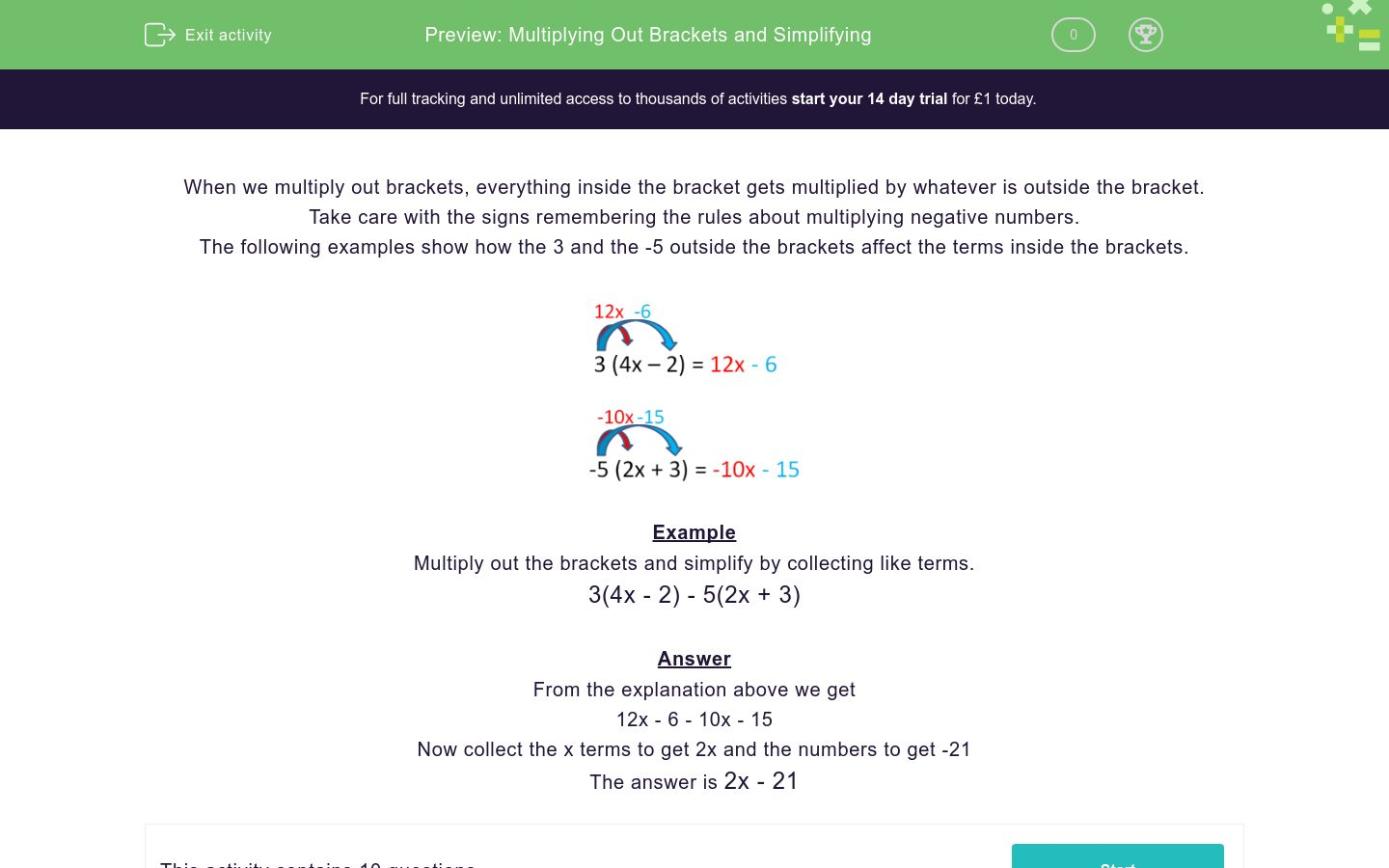# Multiplying Out Brackets and Simplifying

In this worksheet, students multiply out brackets containing algebraic terms and then collect like terms.Key stage:  KS 3

Curriculum topic:   Algebra

Curriculum subtopic:   Simplify Algebraic Expressions to Maintain Equivalence

Difficulty level:### QUESTION 1 of 10

When we multiply out brackets, everything inside the bracket gets multiplied by whatever is outside the bracket.

Take care with the signs remembering the rules about multiplying negative numbers.

The following examples show how the 3 and the -5 outside the brackets affect the terms inside the brackets.Example

Multiply out the brackets and simplify by collecting like terms.

3(4x - 2) - 5(2x + 3)

From the explanation above we get

12x - 6 - 10x - 15

Now collect the x terms to get 2x and the numbers to get -21

The answer is 2x - 21

Multiply out the brackets and simplify by collecting like terms.

3(-2x + 4) + 2(x + 1)

-8x + 14

-4x + 14

32x + 34

Multiply out the brackets and select the correct answer.

3(5x + 14) + 2(x + 1)

35x + 314

17x + 44

15x + 44

Multiply out the brackets and select the correct answer.

3(5x - 40) - 2(x + 1)

35x - 340

13x - 40

13x - 122

Multiply out the brackets and select the correct answer.

30(4x - 3) - 2(2x + 1)

116x - 332

116x - 92

30x - 33

Multiply out the brackets and select the correct answer.

-3(4x - 15) - (x + 1)

-13x + 44

11x - 44

-8x - 19

Multiply out the brackets and select the correct answer.

-3(15x + 9) - 2(x - 1)

-47x - 29

-43x - 29

-47x - 25

Multiply out the brackets and select the correct answer.

-30(5x - 12) - (x - 6)

150x - 48

-151x - 354

-151x + 366

Multiply out the brackets and select the correct answer.

3(15 - 2x) - 3 (x - 2)

45x - 6

9x - 1

51 - 9x

Multiply out the brackets and select the correct answer.

-3(25 - x) - 3(2x + 1)

-78 - 3x

78x - 3

-75 - 3x

Multiply out the brackets and select the correct answer.

-4(25x + 4) + 5(20x - 3)

-200x -31

-31

200x + 1

• Question 1

Multiply out the brackets and simplify by collecting like terms.

3(-2x + 4) + 2(x + 1)

-4x + 14
EDDIE SAYS
Step 1: Expand both brackets -6x + 12 + 2x + 2 Step 2: Collect the alike terms -4x + 14
• Question 2

Multiply out the brackets and select the correct answer.

3(5x + 14) + 2(x + 1)

17x + 44
EDDIE SAYS
Step 1: Expand both brackets 15x + 42 + 2x + 2 Step 2: Collect the alike terms 17x + 44
• Question 3

Multiply out the brackets and select the correct answer.

3(5x - 40) - 2(x + 1)

13x - 122
EDDIE SAYS
Step 1: Expand both brackets 15x - 120 -2x - 2 Step 2: Collect the alike terms 13x - 122
• Question 4

Multiply out the brackets and select the correct answer.

30(4x - 3) - 2(2x + 1)

116x - 92
EDDIE SAYS
Step 1: Expand both brackets 120x - 90 - 4x - 2 Step 2: Collect the alike terms 116x - 92
• Question 5

Multiply out the brackets and select the correct answer.

-3(4x - 15) - (x + 1)

-13x + 44
EDDIE SAYS
Step 1: Expand both brackets -12x + 45 - x - 1 Step 2: Collect the alike terms -13x + 44
• Question 6

Multiply out the brackets and select the correct answer.

-3(15x + 9) - 2(x - 1)

-47x - 25
EDDIE SAYS
Step 1: Expand both brackets -45x - 27 - 2x + 2 Step 2: Collect the alike terms -47x - 25
• Question 7

Multiply out the brackets and select the correct answer.

-30(5x - 12) - (x - 6)

-151x + 366
EDDIE SAYS
Step 1: Expand both brackets -150x + 360 - x + 6 Step 2: Collect the alike terms -151x + 366
• Question 8

Multiply out the brackets and select the correct answer.

3(15 - 2x) - 3 (x - 2)

51 - 9x
EDDIE SAYS
Step 1: Expand both brackets 45 - 6x - 3x + 6 Step 2: Collect the alike terms -9x + 51 or 51 - 9x
• Question 9

Multiply out the brackets and select the correct answer.

-3(25 - x) - 3(2x + 1)

-78 - 3x
EDDIE SAYS
Step 1: Expand both brackets -75 + 3x - 6x - 3 Step 2: Collect the alike terms -3x - 78 or -78 - 3x
• Question 10

Multiply out the brackets and select the correct answer.

-4(25x + 4) + 5(20x - 3)

-31
EDDIE SAYS
Step 1: Expand both brackets -100x - 16 + 100 - 15 Step 2: Collect the alike terms -31
---- OR ----

Sign up for a £1 trial so you can track and measure your child's progress on this activity.

### What is EdPlace?

We're your National Curriculum aligned online education content provider helping each child succeed in English, maths and science from year 1 to GCSE. With an EdPlace account you’ll be able to track and measure progress, helping each child achieve their best. We build confidence and attainment by personalising each child’s learning at a level that suits them.

Get started